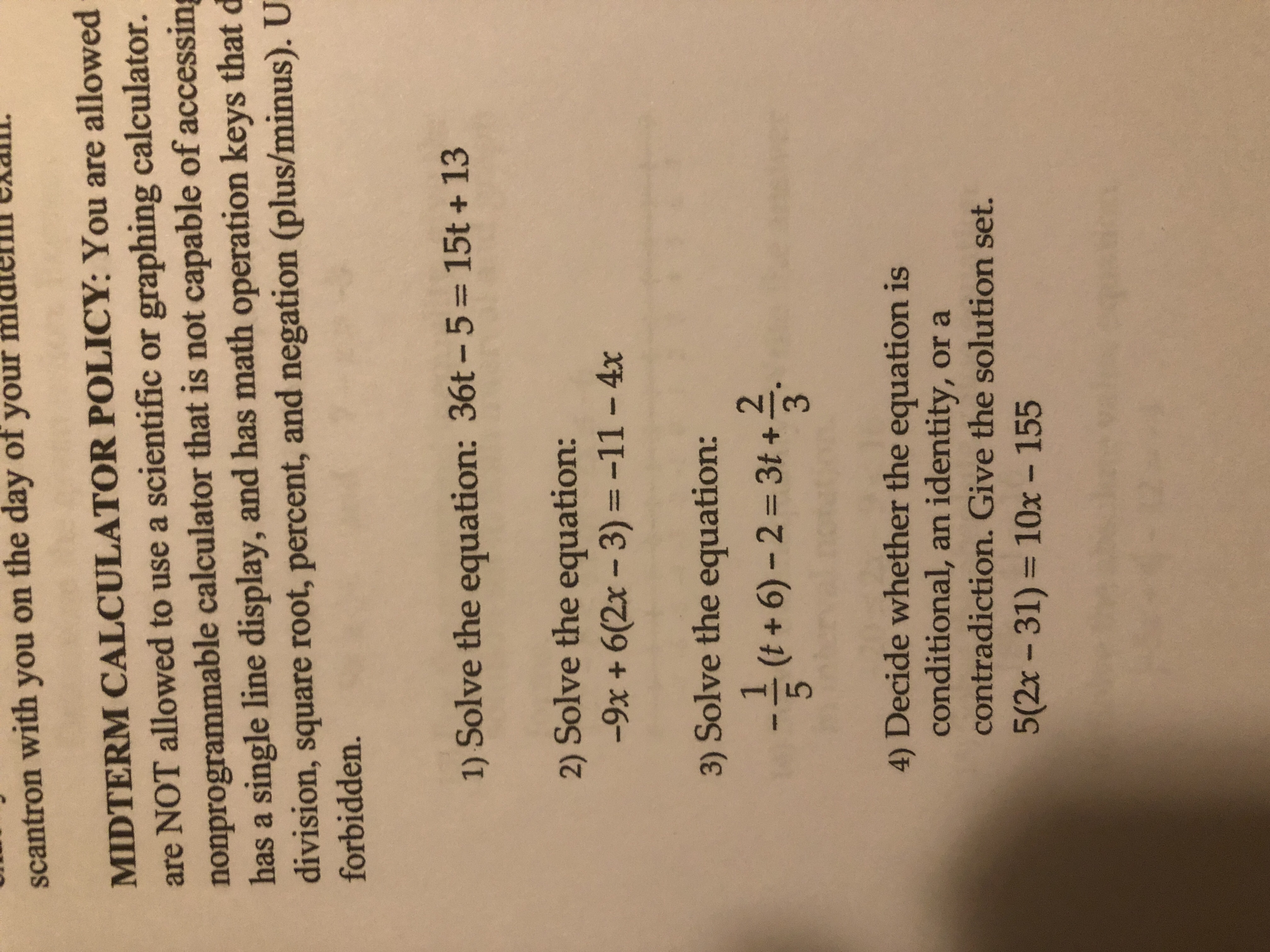# scantron with you on the day of your midterlll exaili MIDTERM CALCULATOR POLICY: You are allowed are NOT allowed to use a scientific or graphing calculator. nonprogrammable calculator that is not capable of accessing has a single line display, and has math operation keys that d division, square root, percent, and negation (plus/minus). U forbidden. 1) Solve the equation: 36t-5 = 15t + 13 2) Solve the equation: -9x + 6(2x -3)-11-4x 3) Solve the equation: 2 3 --" (t + 6)-2-3t + 4) Decide whether the equation is conditional, an identity, or a contradiction. Give the solution set. 5(2x-31) = 10x-155

Question

I need help with the steps to get the answershelp_outlineImage Transcriptionclosescantron with you on the day of your midterlll exaili MIDTERM CALCULATOR POLICY: You are allowed are NOT allowed to use a scientific or graphing calculator. nonprogrammable calculator that is not capable of accessing has a single line display, and has math operation keys that d division, square root, percent, and negation (plus/minus). U forbidden. 1) Solve the equation: 36t-5 = 15t + 13 2) Solve the equation: -9x + 6(2x -3)-11-4x 3) Solve the equation: 2 3 --" (t + 6)-2-3t + 4) Decide whether the equation is conditional, an identity, or a contradiction. Give the solution set. 5(2x-31) = 10x-155 fullscreen

### Want to see the step-by-step answer?